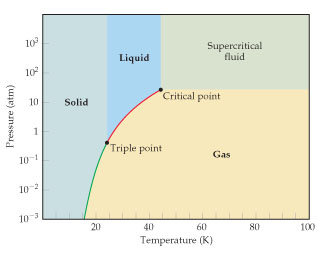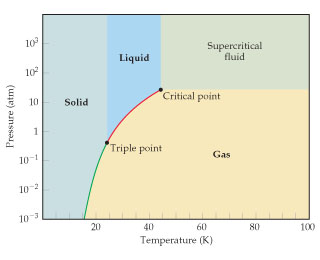# Problem: Use the phase diagram for neon to answer the following questions:At temperature T  =-100 oC can neon be liquefied by compressing it?

###### FREE Expert Solution

This given phase diagram is:93% (95 ratings)###### Problem Details

Use the phase diagram for neon to answer the following questions:At temperature T  =-100 oC can neon be liquefied by compressing it?

Frequently Asked Questions

What scientific concept do you need to know in order to solve this problem?

Our tutors have indicated that to solve this problem you will need to apply the Phase Diagram concept. You can view video lessons to learn Phase Diagram. Or if you need more Phase Diagram practice, you can also practice Phase Diagram practice problems.

What professor is this problem relevant for?

Based on our data, we think this problem is relevant for Professor Davis' class at UCF.